# Support Vector Machines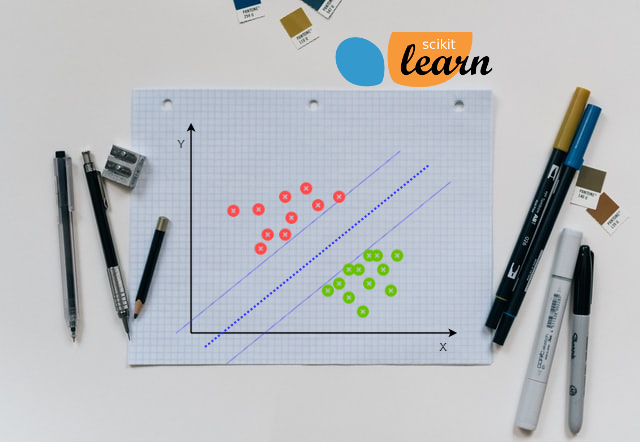Support vector machines (SVM) is one of the most powerful ‘Black Box’ machine learning algorithm. It belongs to the family of supervised learning algorithm. Used to solve classification as well as regression problems. Using threshold to classify the different groups is not very accurate and may lead to the wrong predictions. SVM classifier also use the threshold to classify the data, but it uses midpoint of the observations on the edge of each group as threshold. In order to avoid the classification error, SVM also defines the safety margin on both the side of threshold. So this safety margin will provide robustness to SVM and that’s why it is called as ‘Large Margin Classifier’. SVM algorithm can be used to classify linear as well as non-linear data. So the secret sauce of the SVM is the way it finds the threshold and define the safety margin on either side of threshold to avoid the classification errors.

# How SVM Classifier Work

Outliers in the data can affect the threshold value and lead to wrong predictions. Consider below example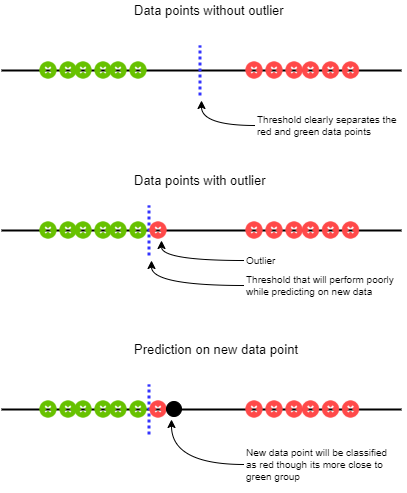So from above example its clear that we can’t just choose data point on edge to draw a decision boundary. In order to avoid this, SVM use cross validation technique to identify the data points to draw the decision boundary. The data points on the edge and within the boundary are called support vectors. Data points inside the margin are also called as ‘misclassified observations’. So SVM using cross validation tries multiple combination of support vectors to find the best decision boundary and finally select the best possible support vector which provides the larger margin. Consider below example where SVM has ignored the few observations in order to find more robust threshold and safety margin.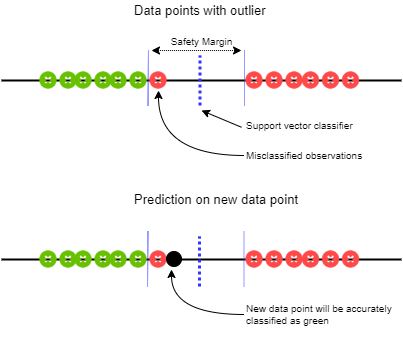## Types Of SVM Classifiers

Since SVM can be used to classify linear as well as non-linear data, we can have support vector classifiers from a point to hyperplane. Hyperplane in this context is tool that separates the data space into one less dimension for easier classification. Actually every SVM classifier is hyperplane of dimension n - 1 where, n is the dimension of given data.

For 1 dimensional data, hyperplane is a point, and we can classify the new observation based on the which side of the point they areFor 2 dimensional data, hyperplane is a line, and we can classify the new observation based on the which side of the line they are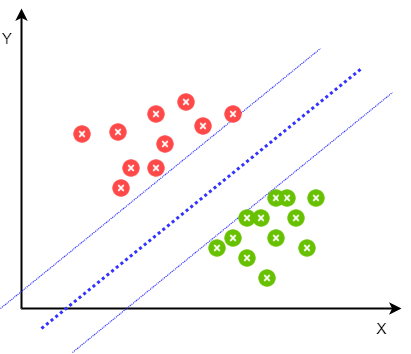For 3 dimensional data, hyperplane is a plane, and we can classify the new observation based on the which side of the plane they are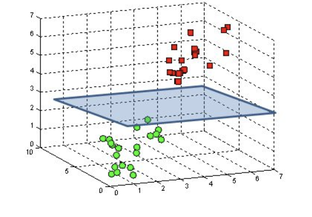For n dimensional data, hyperplane is n-1 dimensional plane

Since we can visualize the data up to 3 dimensions, we refer the hyperplane by more friendly names like line, plane etc and for more than 3 dimensions we just call it hyperplane.

# Kernels

Kernel is the technique used by SVM to classify the non-linear data. Kernel functions are used to increase the dimension of the data, so that SVM can fit the optimum hyperplane to separate the data. Consider below example where 1D data points are randomly grouped. Using kernel function(here 2nd degree polynomial) we can convert 1D data points to 2D data points and fit a line to separate the data into two groups.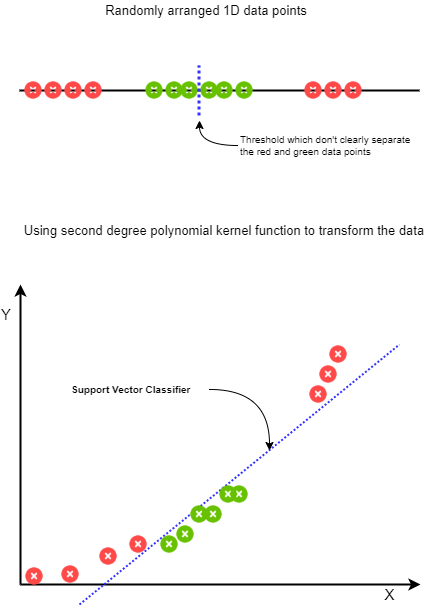So kernel functions help SVM to transform the lower dimension data to higher dimension but in order to figure out which kernel function to use, to transform the data SVM uses cross validation. Using cross validation SVM tries multiple combination of Kernel functions like polynomial kernel and choose the one which results in the best classification.

# Advantages

• Effective in high dimensional input data
• Effective when number of dimensions are higher than number of samples
• Uses a subset of training points to find the support vector classifier, so it is also memory efficient
• Prediction accuracy is higher when there is a clear separation between classes

# Disadvantages

• Large data sets- takes lots of time to separate the data
• Data with lots of error- Since SVM separates the two groups of data based on nearest points, if these points have errors in them, then it will affect entire model performance
• Choose the wrong kernel- If we choose the wrong separation plane then it will affect the model performance

# Example: Classification Problem

Now we will implement the SVM algorithm using sklearn library and build a classification model that estimates an applicant’s probability of admission based on Exam 1 and Exam 2 scores. Note- I have also used the same dataset in Logistic Regression From Scratch With Python

## Import Libraries

• pandas: Used for data manipulation and analysis
• numpy : Numpy is the core library for scientific computing in Python. It is used for working with arrays and matrices.
• matplotlib : It’s plotting library, and we are going to use it for data visualization
• model_selection: Here we are going to use model_selection.train_test_split() for splitting the data
• svm: Sklearn support vector machine model
``````import pandas as pd
import numpy as np
import matplotlib.pyplot as plt
from sklearn import model_selection
from sklearn import svm
``````

## Load Data

• We are going to use ‘admission_basedon_exam_scores.csv’ CSV file
• File contains three columns Exam 1 marks, Exam 2 marks and Admission status
``````df = pd.read_csv('https://raw.githubusercontent.com/satishgunjal/datasets/master/admission_basedon_exam_scores.csv')
print('Shape of data= ', df.shape)
df.head()
``````
``````Shape of data=  (100, 3)
``````
Exam 1 marks Exam 2 marks Admission status
0 34.623660 78.024693 0
1 30.286711 43.894998 0
2 35.847409 72.902198 0
3 60.182599 86.308552 1
4 79.032736 75.344376 1

## Data Understanding

• There are total 100 training examples (m= 100 or 100 no of rows)
• There are two features Exam 1 marks and Exam 2 marks
• Label column contains application status. Where ‘1’ means admitted and ‘0’ means not admitted

### Data Visualization

To plot the data of admitted and not admitted applicants, we need to first create separate data frame for each class(admitted/not-admitted)

``````df_admitted = df[df['Admission status'] == 1]
print('Training examples with admission status 1 are = ', df_admitted.shape)
df_admitted.head(3)
``````
``````Training examples with admission status 1 are =  60
``````
Exam 1 marks Exam 2 marks Admission status
3 60.182599 86.308552 1
4 79.032736 75.344376 1
6 61.106665 96.511426 1
``````df_notadmitted = df[df['Admission status'] == 0]
print('Training examples with admission status 0 are = ', df_notadmitted.shape)
df_notadmitted.head(3)
``````
``````Training examples with admission status 0 are =  40
``````
Exam 1 marks Exam 2 marks Admission status
0 34.623660 78.024693 0
1 30.286711 43.894998 0
2 35.847409 72.902198 0

Now lets plot the scatter plot for admitted and not admitted students

``````def plot_data(title):
plt.figure(figsize=(10,6))
plt.scatter(df_admitted['Exam 1 marks'], df_admitted['Exam 2 marks'], color= 'green', label= 'Admitted Applicants')
plt.scatter(df_notadmitted['Exam 1 marks'], df_notadmitted['Exam 2 marks'], color= 'red', label= 'Not Admitted Applicants')
plt.xlabel('Exam 1 Marks')
plt.ylabel('Exam 2 Marks')
plt.title(title)
plt.legend()

plot_data(title = 'Admitted Vs Not Admitted Applicants')
``````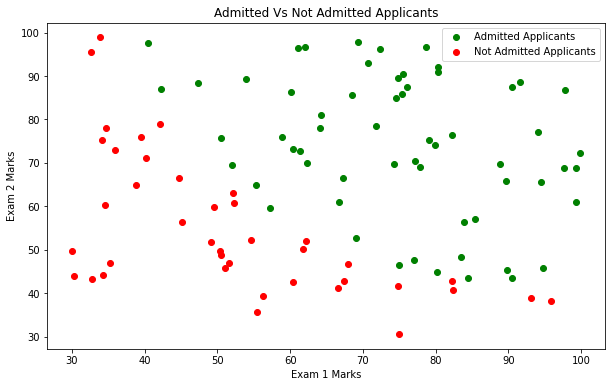## Build Machine Learning Model

``````#Lets create feature matrix X and label vector y
X = df[['Exam 1 marks', 'Exam 2 marks']]
y = df['Admission status']

print('Shape of X= ', X.shape)
print('Shape of y= ', y.shape)
``````
``````Shape of X=  (100, 2)
Shape of y=  (100,)
``````

### Create Test And Train Dataset

• We will split the dataset, so that we can use one set of data for training the model and one set of data for testing the model
• We will keep 20% of data for testing and 80% of data for training the model
• If you want to learn more about it, please refer Train Test Split tutorial
``````X_train, X_test, y_train, y_test = model_selection.train_test_split(X, y, test_size=0.2, random_state= 1)

print('X_train dimension= ', X_train.shape)
print('X_test dimension= ', X_test.shape)
print('y_train dimension= ', y_train.shape)
print('y_train dimension= ', y_test.shape)
``````
``````X_train dimension=  (80, 2)
X_test dimension=  (20, 2)
y_train dimension=  (80,)
y_train dimension=  (20,)
``````

Now lets train the model using SVM classifier

``````# Note here we are using default SVC parameters
clf = svm.SVC()
clf.fit(X_train, y_train)
print('Model score using default parameters is = ', clf.score(X_test, y_test))
``````
``````Model score using default parameters is =  0.85
``````

In order to visualize the results better lets create a function to plot SVM Classifier decision boundary with margin

``````def plot_support_vector(classifier):
"""
To plot decsion boundary and margin. Code taken from Sklearn documentation.

I/P
----------
classifier : SVC object for each type of kernel

O/P
-------
Plot

"""
clf =classifier
# plot the decision function
ax = plt.gca()
xlim = ax.get_xlim()
ylim = ax.get_ylim()

# create grid to evaluate model
xx = np.linspace(xlim, xlim, 30)
yy = np.linspace(ylim, ylim, 30)
YY, XX = np.meshgrid(yy, xx)
xy = np.vstack([XX.ravel(), YY.ravel()]).T
Z = clf.decision_function(xy).reshape(XX.shape)

# plot decision boundary and margins
ax.contour(XX, YY, Z, colors='k', levels=[-1, 0, 1], alpha=0.5,
linestyles=['--', '-', '--'])
# plot support vectors
ax.scatter(clf.support_vectors_[:, 0], clf.support_vectors_[:, 1], s=100,
linewidth=1, facecolors='none', edgecolors='k')
``````
``````plot_data(title = 'SVM Classifier With Default Parameters')
plot_support_vector(clf)
``````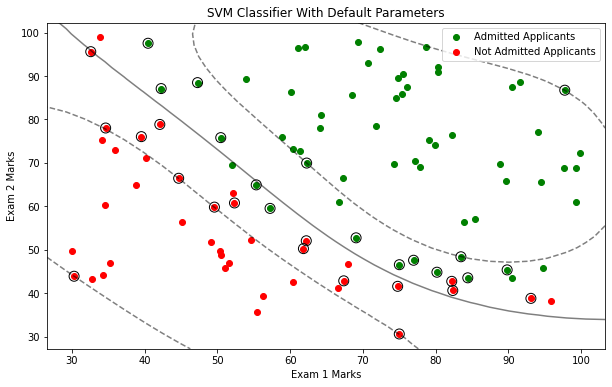## SVM Parameters

• Gamma: In case of high value of Gamma decision boundary is dependent on observations close to it, where in case of low value of Gamma, SVM will consider the far away points also while deciding the decision boundary
• Regularization parameter(C): Large C will result in overfitting and which will lead to lower bias and high variance. Small C will result in underfitting and which will lead to higher bias and low variance. For more details about it please refer Underfitting & Overfitting

So regularization parameter C and gamma parameters plays an important role in order to find the best fit model. Let’s create a function which will try multiple such values and return the best value of C and gamma for our choice of the kernel. At the end we will plot the decision boundary with margin using the best choice of SVM parameters for each type of kernel.

``````def svm_params(X_train, y_train, X_test, y_test):
"""
Finds the best choice of Regularization parameter (C) and gamma for given choice of kernel and returns the SVC object for each type of kernel

I/P
----------
X_train : ndarray
Training samples
y_train : ndarray
Labels for training set
X_test : ndarray
Test data samples
y_test : ndarray
Labels for test set.

O/P
-------
classifiers : SVC object for each type of kernel

"""
C_values = [0.01, 0.03, 0.1, 0.3, 1, 3, 10, 30, 40]
gamma_values = [0.01, 0.03, 0.1, 0.3, 1, 3, 10, 30, 40]
kernel_types = ['linear', 'poly', 'rbf']
classifiers = {}
max_score = -1
C_final = -1
gamma_final = -1
for kernel in kernel_types:
for C in C_values:
for gamma in gamma_values:
clf = svm.SVC(C=C, kernel= kernel, gamma=gamma)
clf.fit(X_train, y_train)
score = clf.score(X_test, y_test)
#print('C = %s, gamma= %s, score= %s' %(C, gamma, score))
if score > max_score:
max_score = score
C_final = C
gamma_final = gamma
classifiers[kernel] = clf
print('kernel = %s, C = %s, gamma = %s, score = %s' %(kernel, C_final, gamma_final, max_score))
return classifiers
``````

Lets call the svm_params() function to get the best parameters for each type of kernel

``````classifiers = svm_params(X_train, y_train, X_test, y_test)
``````
``````kernel = linear, C = 0.01, gamma = 0.01, score = 0.85
kernel = poly, C = 0.01, gamma = 0.01, score = 0.95
kernel = rbf, C = 1, gamma = 0.03, score = 1.0
``````
``````plot_data(title = 'SVM Classifier With Parameters ' + str(classifiers['linear']))
plot_support_vector(classifiers['linear'])
``````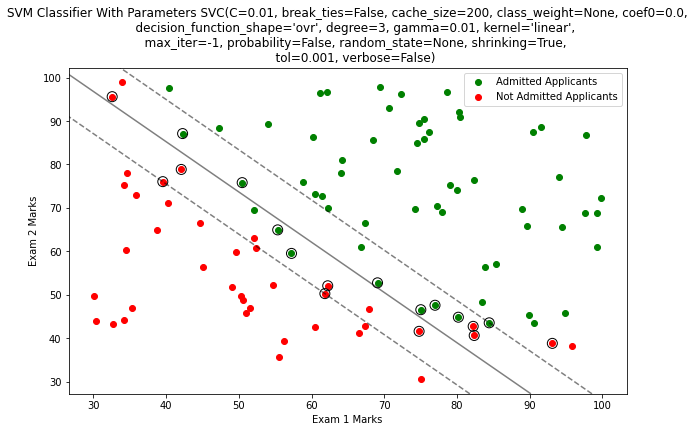``````plot_data(title = 'SVM Classifier With Parameters ' + str(classifiers['rbf']))
plot_support_vector(classifiers['rbf'])
``````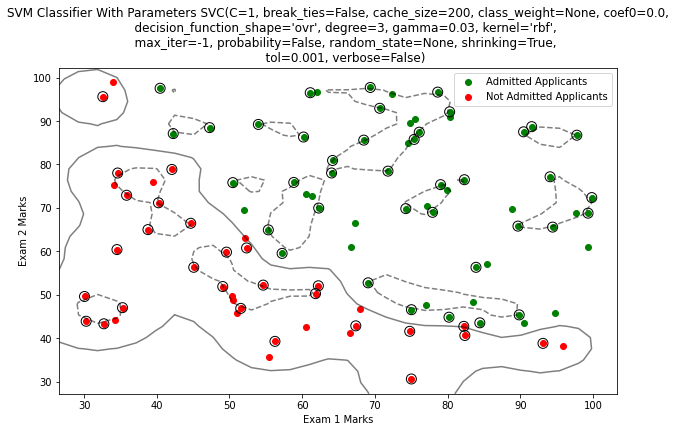``````plot_data(title = 'SVM Classifier With Parameters ' + str(classifiers['poly']))
plot_support_vector(classifiers['poly'])
``````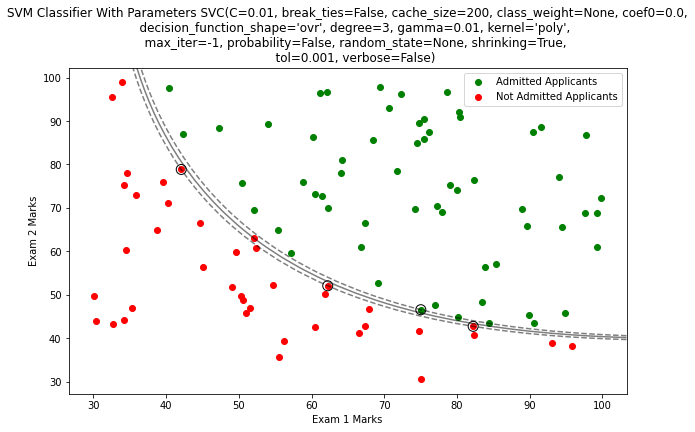# Conclusion

Remember that, our data is 2D so hyperplane will be a line. But if you observe the data closely there is no clear separation between classes that’s why straight line is not a good fit, which is obvious from above plots. Though the accuracy of poly kernel is less than rbf, but still its best choice for our data.

## Learning Path for DP-900 Microsoft Azure Data Fundamentals Certification

8 minute read

Learning path to gain necessary skills and to clear the Azure Data Fundamentals Certification. This certification is intended for candidates beginning to wor...

## Learning Path for AI-900 Microsoft Azure AI Fundamentals Certification

7 minute read

Learning path to gain necessary skills and to clear the Azure AI Fundamentals Certification. This certification is intended for candidates with both technica...

## ANN Model to Classify Images

12 minute read

In this guide we are going to create and train the neural network model to classify the clothing images. We will use TensorFlow deep learning framework along...

## Introduction to NLP

8 minute read

In short NLP is an AI technique used to do text analysis. Whenever we have lots of text data to analyze we can use NLP. Apart from text analysis, NLP also us...

## K Fold Cross Validation

14 minute read

There are multiple ways to split the data for model training and testing, in this article we are going to cover K Fold and Stratified K Fold cross validation...

## K-Means Clustering

13 minute read

K-Means clustering is most commonly used unsupervised learning algorithm to find groups in unlabeled data. Here K represents the number of groups or clusters...

## Time Series Analysis and Forecasting

10 minute read

Any data recorded with some fixed interval of time is called as time series data. This fixed interval can be hourly, daily, monthly or yearly. Objective of t...

## Support Vector Machines

9 minute read

Support vector machines is one of the most powerful ‘Black Box’ machine learning algorithm. It belongs to the family of supervised learning algorithm. Used t...

## Random Forest

11 minute read

Random forest is supervised learning algorithm and can be used to solve classification and regression problems. Unlike decision tree random forest fits multi...

## Decision Tree

14 minute read

Decision tree explained using classification and regression example. The objective of decision tree is to split the data in such a way that at the end we hav...

## Agile Scrum Framework

7 minute read

This tutorial covers basic Agile principles and use of Scrum framework in software development projects.

## Underfitting & Overfitting

2 minute read

Main objective of any machine learning model is to generalize the learning based on training data, so that it will be able to do predictions accurately on un...

## Multiclass Logistic Regression Using Sklearn

6 minute read

In this study we are going to use the Linear Model from Sklearn library to perform Multi class Logistic Regression. We are going to use handwritten digit’s d...

## Binary Logistic Regression Using Sklearn

5 minute read

In this tutorial we are going to use the Logistic Model from Sklearn library. We are also going to use the same test data used in Logistic Regression From Sc...

## Logistic Regression From Scratch With Python

13 minute read

This tutorial covers basic concepts of logistic regression. I will explain the process of creating a model right from hypothesis function to algorithm. We wi...

## Train Test Split

3 minute read

In this tutorial we are going to study about train, test data split. We will use sklearn library to do the data split.

## One Hot Encoding

11 minute read

In this tutorial we are going to study about One Hot Encoding. We will also use pandas and sklearn libraries to convert categorical data into numeric data.

## Multivariate Linear Regression Using Scikit Learn

8 minute read

In this tutorial we are going to use the Linear Models from Sklearn library. Scikit-learn is one of the most popular open source machine learning library for...

## Univariate Linear Regression Using Scikit Learn

7 minute read

In this tutorial we are going to use the Linear Models from Sklearn library. Scikit-learn is one of the most popular open source machine learning library for...

## Multivariate Linear Regression From Scratch With Python

10 minute read

In this tutorial we are going to cover linear regression with multiple input variables. We are going to use same model that we have created in Univariate Lin...

## Univariate Linear Regression From Scratch With Python

13 minute read

This tutorial covers basic concepts of linear regression. I will explain the process of creating a model right from hypothesis function to gradient descent a...

## Machine Learning Introduction And Learning Plan

4 minute read

In this tutorial we will see the brief introduction of Machine Learning and preferred learning plan for beginners

Back to top ↑